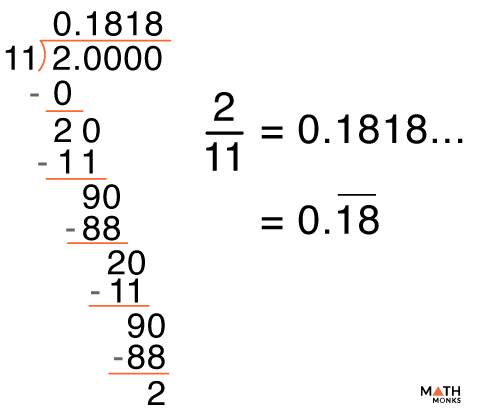# Non Terminating Decimal

Non-terminating decimals are decimal numbers that do not have an end term. We get such decimals when we divide a dividend by a divisor. However, the remainder is never zero, and the division continues forever. The digits are infinite after the decimal point in such decimals.

An example is shown below with the expansion:

We can further divide non-terminating decimals into two categories:

## Non-Terminating Repeating Decimals

The decimal numbers that continue forever with a digit (or group of digits) repeating periodically. Such decimals are also called recurring or repeating decimals. These are always rational numbers.  E.g., 15/7 = 2.142857142857…

### Converting to Rational Numbers

Suppose we are given a repeating decimal, i.e., 0.5555…

We can write it as ${ 0.\overline{5}}$

Let us convert the decimal to fraction.

Let, x = 0.5555… ——- (1)

Multiplying 10 on both sides,

10x = 5.5555… ——- (2) (this is done to eliminate the digits after the decimal point)

Subtracting (1) from (2)

10x – x = 5.5555… – 0.5555…

9x = 5

x = 5/9

∴${ 0.\overline{5}}$ = ${\dfrac{5}{9}}$

Let us take another example of a mixed repeating decimal (where a group of digits is repeated periodically).

Given a decimal 0.7575…

We can write it as ${ 0.\overline{75}}$

Now, let, x = 0.7575… ——- (1)

Multiplying 100 on both sides,

100x = 75.7575… ——- (2)

Subtracting (1) from (2)

100x – x = 75.7575 – 0.7575

99x = 75

x = 75/99

## Non-Terminating Non-Repeating Decimals

The decimal numbers that continue forever with no digit (or group of digits) repeating. Such decimals are also called non-recurring or non-repeating decimals. These are always irrational numbers. E.g., π = 3.141592653…, √2 = 1.4142135623…, 737.537269541…, or 1.41421356237309504….

## Solved Examples

Represent 2/11 by its expansion. Write the type of decimal from the result.Solution:

The division is shown in the figure along side.
From the solution, we see that 18 is being repeated in period of 2.
So the decimal can be specifically said to be non-terminating repeating decimal.

Q1. Non-terminating decimals are rational numbers. True or False

Ans. False. Non-terminating decimals are irrational numbers.